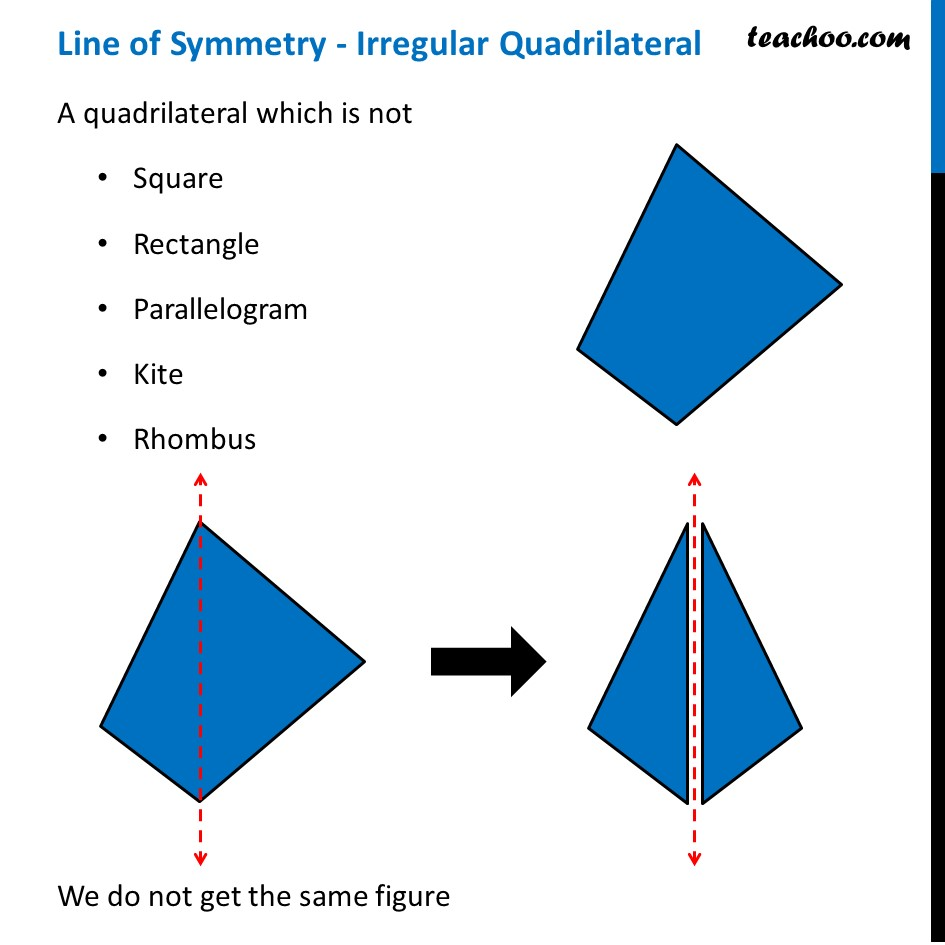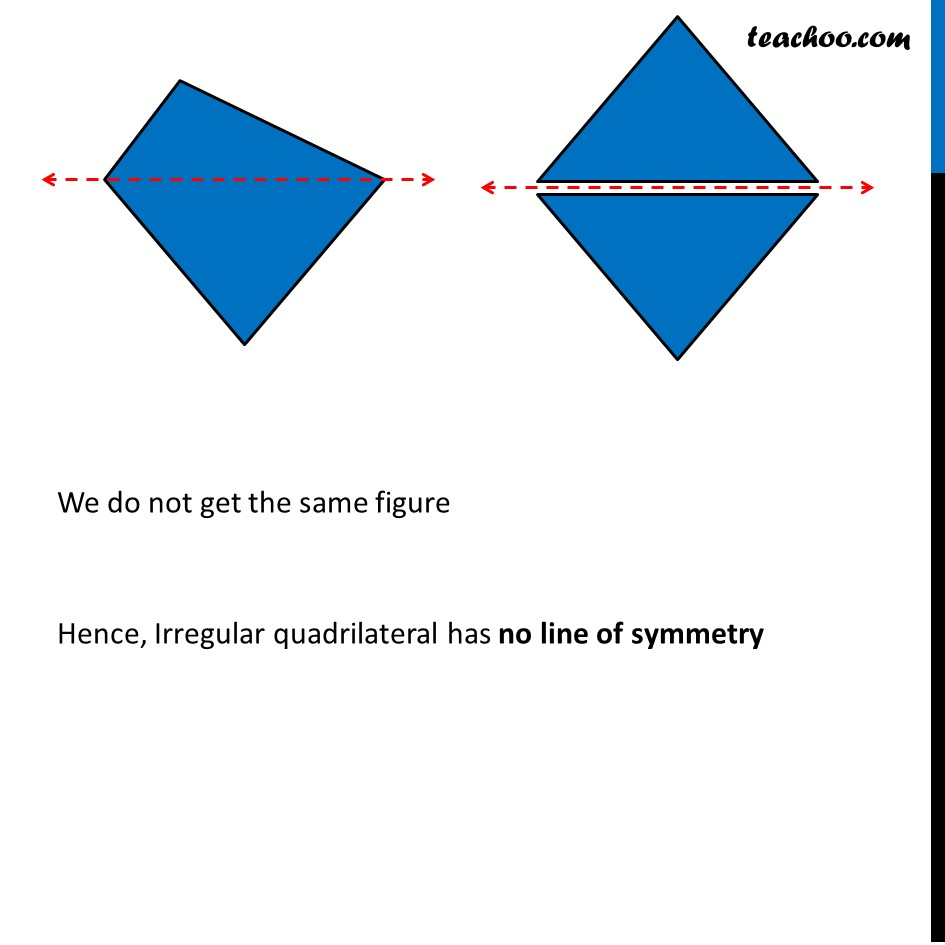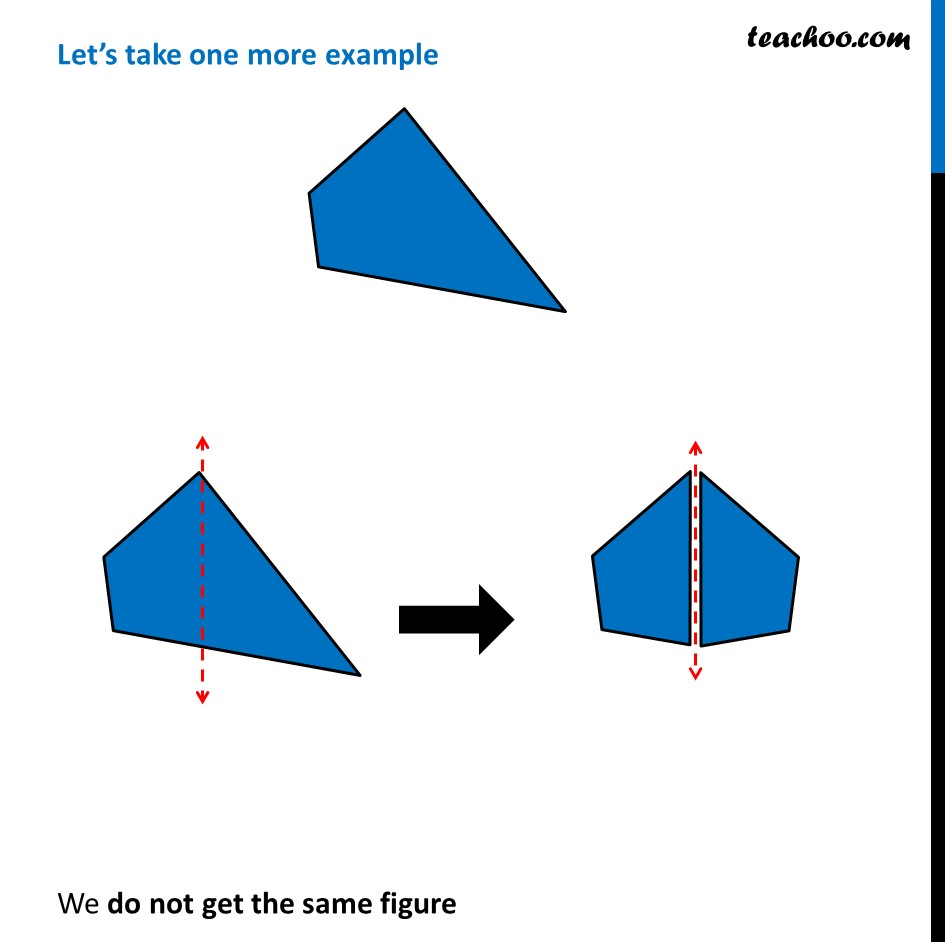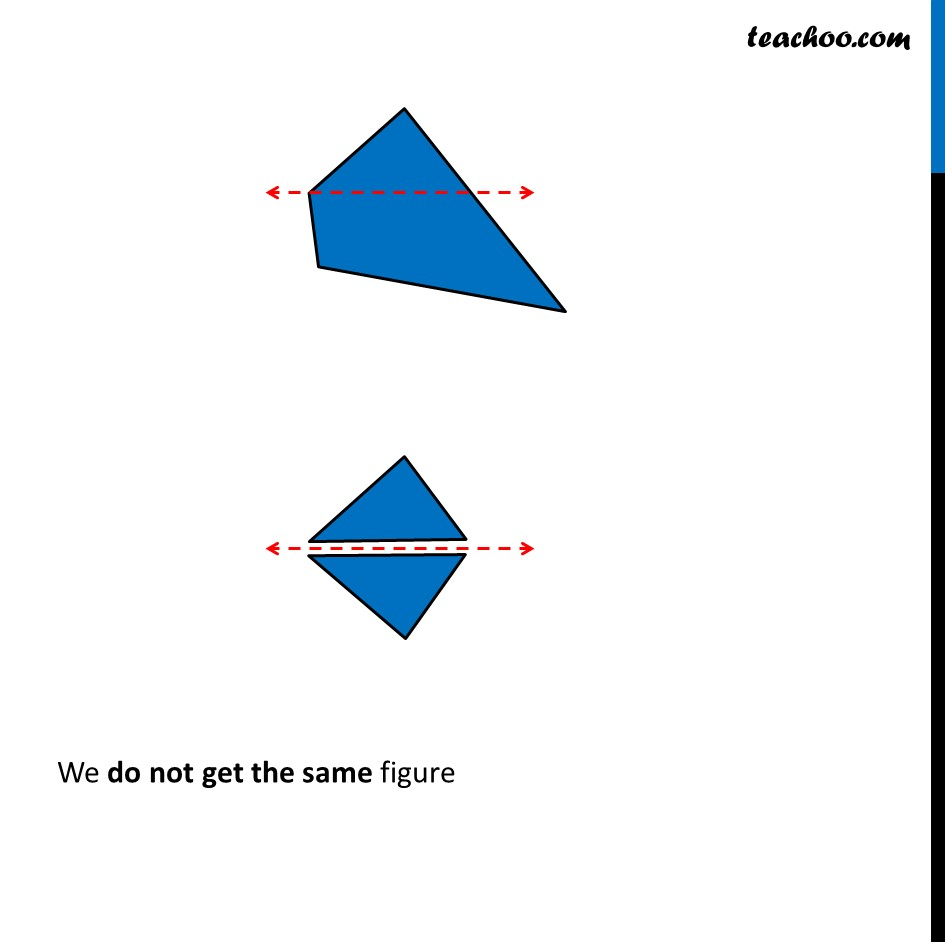1. Chapter 13 Class 6 Symmetry
2. Concept wise
3. Figures with more than one lines of symmetry

Transcript

Line of Symmetry - Irregular Quadrilateral A quadrilateral which is not Square Rectangle Parallelogram Kite Rhombus We do not get the same figure We do not get the same figure Hence, Irregular quadrilateral has no line of symmetry Let’s take one more example We do not get the same figure We do not get the same figure We do not get the same figure

Figures with more than one lines of symmetry

Chapter 13 Class 6 Symmetry
Concept wise

About the AuthorDavneet Singh
Davneet Singh is a graduate from Indian Institute of Technology, Kanpur. He has been teaching from the past 10 years. He provides courses for Maths and Science at Teachoo.